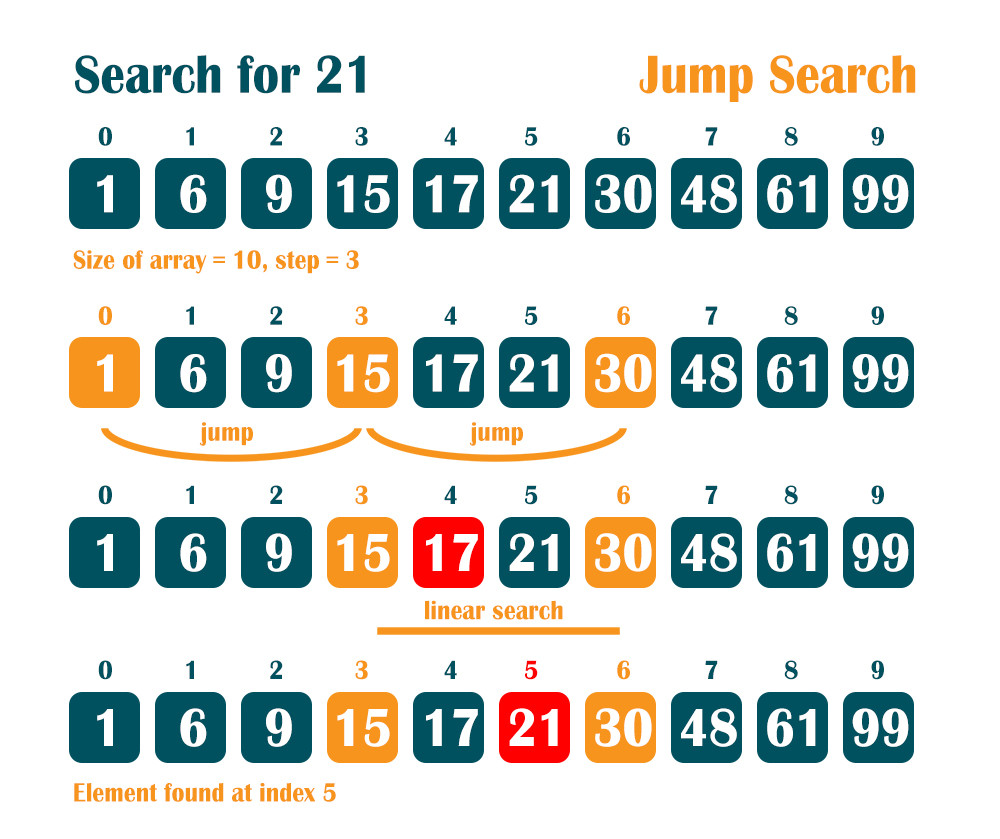Caută
•oanaunciuleanu

# Jump Search in C++Jump Search is an algorithm used to find a value in an ordered array of elements. Its main purpose is to do as few searches as possible. In order to do that it starts from the beginning of the array and it does not check all the values, it jumps with a fixed step from the first element, to the end. If the step is four, it compares the searched value with element on index 0 and then on index 4, then on index 8, and then on index 12, and so on, until the value on the jumped element will be higher than the searched element. At that point we will do a linear search to find the value that interests us. If the size of the array is m, an optimal step value is √m.

```// Jump Search

#include <bits/stdc++.h>
using namespace std;

int jumpSearch(int arrayOfNumbers[], int searchForNumber, int numberOfElements)
{
// The step to jump
int currentStepIndex = sqrt(numberOfElements);
int initialStep = sqrt(numberOfElements);

int currentPosition = 0;
while (arrayOfNumbers[min(currentStepIndex, numberOfElements)-1] < searchForNumber)
{
currentPosition = currentStepIndex;
currentStepIndex += initialStep;
if (currentPosition >= numberOfElements)
{
return -1;
}
}

// A linear search in the last block
while (arrayOfNumbers[currentPosition] < searchForNumber)
{
currentPosition++;

if (currentPosition == min(currentStepIndex, numberOfElements))
{
return -1;
}
}
// When the element is found
if (arrayOfNumbers[currentPosition] == searchForNumber)
{
printf("The number %d is found at index %d", searchForNumber, currentPosition);
return currentPosition;
}

return -1;
}

int main()
{
int arrayOfNumbers[] = {1, 6, 9, 15, 17, 21, 30, 48, 61, 99};
int searchForNumber = 61;
int numberOfElements = sizeof(arrayOfNumbers) / sizeof(arrayOfNumbers);

int result = jumpSearch(arrayOfNumbers, searchForNumber, numberOfElements);
return 0;
}```

65 afișare0 comentariu

## Postări recente

Afișează-le pe toate